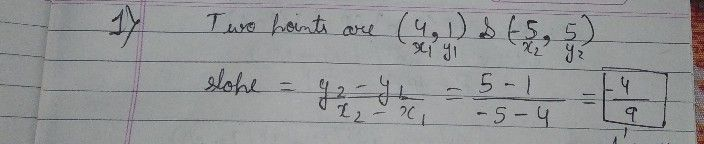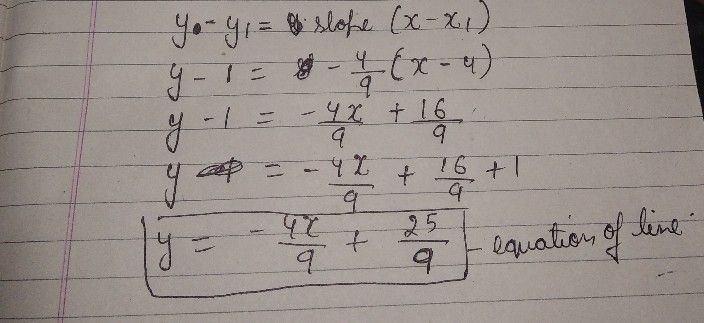Symbol
ProblemLearning Task $c1$ Do the following in your notebook. $1$ Find the equation of the line given the graph. $1$ $2$ $3$ $3$ $2$ $1$ $-0$ $1$ $1$ $11$ $p$ $1$ $3$
7th-9th grade
Algebra
SolutionQanda teacher - Adarshany doubt ask meStudent
your so very very fucking idiot
you are raw shit